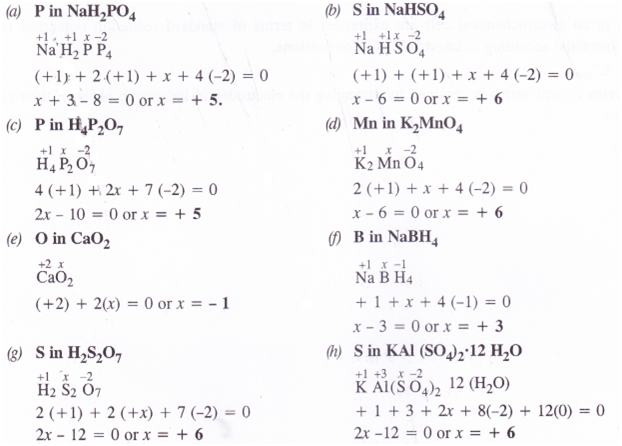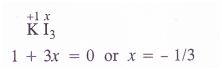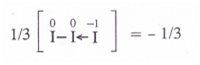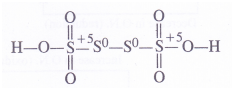• +91 9971497814
• info@interviewmaterial.com

# Chapter 8- Redox Reactions Interview Questions Answers

### Related Subjects

Question 1 : Assign oxidation numbers to the underlined elements ineach of the following species ;
(a) NaH2PO4
(b) NaHSO4
(c) H4P2O7
(d) K2MnO4
(e) CaO2
(f) NaBH4
(g) H2S2O7
(h) KAl(SO4)2.12H2O

Answer 1 :Question 2 : What are the oxidation numbers of the underlinedelements in each of the following and how do you rationalise your results ?|
(a) KI(b) H2S4Oe (c) Fe3O4 (d) CH3CH2OH (e) CH3COOH

Answer 2 : (a) O.N. of iodine in KI3 : By conventional method, the oxidation number of iodine in the I3_ ionmay be expressed as :Explanation : The oxidationnumber of iodine (I) comes out to be fractional which does not seem to bepossible. Let us consider the structure of I3~ ion. In it, two atomsof iodine are linked to each other by covalent bond (I—I). The iodide ion (I~)is linked to the molecule by co-ordinate bond [I—I <—I]“. The molecule maybe represented as K+[I—I <—1| . Now, in the anion, the oxidationnumber of the two I atoms is zero while I ion has – 1oxidation number. The overall oxidation number of L“ ion is :(b) O.N. of S in H2S4O6 :By conventional method, the oxidation number of sulphur may be calculated as :
H+12  SxO-26
2 + 4x + 6 (- 2) =0 or 4x = 12 – 2 = 10 or x = 5/2
Explanation : In order toaccount for the fractional value, let us assign oxidation numbers to differentsulphur atoms in the structural formula of the acid. The oxidation numbers ofthe two middle sulphur atoms is zero while the two atoms at the terminalpositions have + 5 oxidation number.Average O.N. of S =—1/4 [5+ 0 + 0 +5] = 5/2

(c) O. N. of Fe in Fe3O: Byconventional method, oxidation number of Fe may be calculated as :
Fe3x 3 034
3x + 4(- 2) =0 or x = 8/3
Explanation : Fe3O4 isa mixed oxide and is an equimolar mixture of Fe+2 O-2 andFe+32 O-23
Average O.N. of Fe = 1/3(2 + 2 x 3) =8/3

(d) O.N. of C in CH3CH2OH : Byconventional method, the oxidation number of carbon in ethanol molecule may becalculated as :
CH3cH2OH or Cx2 H+16 O-2
2x + 6(+ 1) + (- 2) = 0 or 2x + 6 – 2 = 0 or 2x = – 4 or x = – 2

Explanation : Let uscalculate the oxidation number of both C1 and C2 atoms.
C2 : Thecarbon atom is attached to three H atoms (less electronegative than carbon) andone CH2OH group (more electronegative than carbon).
O.N. of C2 = 3(+ 1) +x + (- 1) = O or x =-2
C1 : The carbon atom isattached to one OH group (O.N. = – 1), two H atoms (O.N. = + 1) and one CH3 group(O.N. = + 1)
O.N. of C1 = ( + 1) + x + 2 (+ 1) + 1(- 1) =0 or x = – 2 Average O.N. of C =l/2[ – 2 + (- 2)] = – 2

(e) O.N. of C in CH3COOH : By conventionalmethod the O.N. of carbon may be calculated as:
CH3COOH or c2 H4 62
2x + 4(+ 1) + 2(— 2) = 0 or x — 0
Explanation : Let uscalculate the oxidation number of both C2 and C1 atoms.
C1 : The carbon atom isattached to three H atoms (less electronegative than carbon) and one COOH group(more electronegative than carbon).
O.N. of C2 =3 (+ 1) + x + 1(- 1) = 0 or x = – 2
C2 : Thecarbon atom is attached to one OH group (O.N. = – 1) one oxygen atom by doublebond
(O.N. = – 2) and one CH3 group (O.N. = + 1)
O.N. of C1 = 1( + 1) + Jt + 1 (-2) + 1(- 1) = O or x = + 2
Average O.N. of C =1/2 [+ 2 + (- 2)] = 0

Question 3 : How will you justify that the following reactionsare redox reactions in nature ?
(a) CuO(s) + H2(g)  → Cu(s) + H2O(g)
(b) Fe2O3(s) + 3CO(g) →2Fe(s) + 3CO2(g)
(c) 2K(s) + F2(g)→2K+F(s)
(d) 4NH3(g) + 5O2(g) → 4NO(g) + 6H2O(g)

Answer 3 : A chemical reaction may be regarded as redox reactionif one of the reacting species undergoes increase in O.N. (oxidation) and theother decrease in O.N. (reduction). Based upon this, let us try to justify thatthe reactions under study are redox reactions in nature.